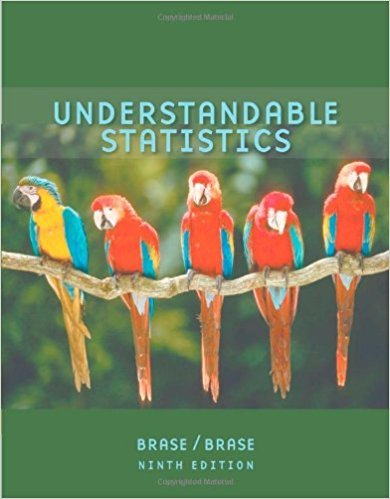×
×

# Solutions for Chapter 1: Getting Started## Full solutions for Understandable Statistics | 9th Edition

ISBN: 9780618949922Solutions for Chapter 1: Getting Started

Solutions for Chapter 1
4 5 0 376 Reviews
16
0
##### ISBN: 9780618949922

This textbook survival guide was created for the textbook: Understandable Statistics, edition: 9. Chapter 1: Getting Started includes 9 full step-by-step solutions. This expansive textbook survival guide covers the following chapters and their solutions. Since 9 problems in chapter 1: Getting Started have been answered, more than 33456 students have viewed full step-by-step solutions from this chapter. Understandable Statistics was written by and is associated to the ISBN: 9780618949922.

Key Statistics Terms and definitions covered in this textbook
• `-error (or `-risk)

In hypothesis testing, an error incurred by rejecting a null hypothesis when it is actually true (also called a type I error).

• Additivity property of x 2

If two independent random variables X1 and X2 are distributed as chi-square with v1 and v2 degrees of freedom, respectively, Y = + X X 1 2 is a chi-square random variable with u = + v v 1 2 degrees of freedom. This generalizes to any number of independent chi-square random variables.

• Average

See Arithmetic mean.

• Binomial random variable

A discrete random variable that equals the number of successes in a ixed number of Bernoulli trials.

• Central composite design (CCD)

A second-order response surface design in k variables consisting of a two-level factorial, 2k axial runs, and one or more center points. The two-level factorial portion of a CCD can be a fractional factorial design when k is large. The CCD is the most widely used design for itting a second-order model.

• Chi-square test

Any test of signiicance based on the chi-square distribution. The most common chi-square tests are (1) testing hypotheses about the variance or standard deviation of a normal distribution and (2) testing goodness of it of a theoretical distribution to sample data

• Conidence coeficient

The probability 1?a associated with a conidence interval expressing the probability that the stated interval will contain the true parameter value.

• Control chart

A graphical display used to monitor a process. It usually consists of a horizontal center line corresponding to the in-control value of the parameter that is being monitored and lower and upper control limits. The control limits are determined by statistical criteria and are not arbitrary, nor are they related to speciication limits. If sample points fall within the control limits, the process is said to be in-control, or free from assignable causes. Points beyond the control limits indicate an out-of-control process; that is, assignable causes are likely present. This signals the need to ind and remove the assignable causes.

• Cumulative distribution function

For a random variable X, the function of X deined as PX x ( ) ? that is used to specify the probability distribution.

• Defect

Used in statistical quality control, a defect is a particular type of nonconformance to speciications or requirements. Sometimes defects are classiied into types, such as appearance defects and functional defects.

• Defect concentration diagram

A quality tool that graphically shows the location of defects on a part or in a process.

• Deming

W. Edwards Deming (1900–1993) was a leader in the use of statistical quality control.

• Error of estimation

The difference between an estimated value and the true value.

• Fixed factor (or fixed effect).

In analysis of variance, a factor or effect is considered ixed if all the levels of interest for that factor are included in the experiment. Conclusions are then valid about this set of levels only, although when the factor is quantitative, it is customary to it a model to the data for interpolating between these levels.

• Fraction defective

In statistical quality control, that portion of a number of units or the output of a process that is defective.

• Frequency distribution

An arrangement of the frequencies of observations in a sample or population according to the values that the observations take on

• Gamma random variable

A random variable that generalizes an Erlang random variable to noninteger values of the parameter r

• Generating function

A function that is used to determine properties of the probability distribution of a random variable. See Moment-generating function

• Goodness of fit

In general, the agreement of a set of observed values and a set of theoretical values that depend on some hypothesis. The term is often used in itting a theoretical distribution to a set of observations.

• Hat matrix.

In multiple regression, the matrix H XXX X = ( ) ? ? -1 . This a projection matrix that maps the vector of observed response values into a vector of itted values by yˆ = = X X X X y Hy ( ) ? ? ?1 .

×